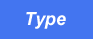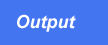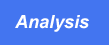# In Review

By now, you know enough about programming in Perl to write programs that perform many useful tasks. The program in Listing R1.1, which takes a number and prints out its English equivalent, illustrates some of the concepts you've learned during first chapters.Listing R1.1. Printing the English equivalent of numeric input.
```
1:  #!/usr/local/bin/perl

2:

3:  # define the strings used in printing

4:  @digitword = ("", "one", "two", "three", "four", "five",

5:       "six", "seven", "eight", "nine");

6:  @digit10word = ("", "ten", "twenty", "thirty", "forty",

7:       "fifty", "sixty", "seventy", "eighty", "ninety");

8:  @teenword = ("ten", "eleven", "twelve", "thirteen", "fourteen",

9:      "fifteen", "sixteen", "seventeen", "eighteen", "nineteen");

10: @groupword = ("", "thousand", "million", "billion", "trillion",

12:     "octillion", "novillion", "decillion");

13:

14: # read a line of input and remove all blanks, commas and tabs;

15: # complain about anything else

16: \$inputline = <STDIN>;

17: chop (\$inputline);

18: \$inputline =~ s/[, \t]+//g;

19: if (\$inputline =~ /[^\d]/) {

20:          die ("Input must be a number.\n");

21: }

22:

24: \$inputline =~ s/^0+//;

25: \$inputline =~ s/^\$/0/;  # put one back if they're all zero

26:

27: # split into digits: \$grouping contains the number of groups

28: # of digits, and \$oddlot contains the number of digits in the

29: # first group, which may be only 1 or 2 (e.g., the 1 in 1,000)

30: @digits = split(//, \$inputline);

31: if (@digits > 36) {

32:         die ("Number too large for program to handle.\n");

33: }

34: \$oddlot = @digits % 3;

35: \$grouping = (@digits-1) / 3;

36:

37: # this loop iterates once for each grouping

38: \$count = 0;

39: while (\$grouping >= 0) {

40:         if (\$oddlot == 2) {

41:                 \$digit1 = 0;

42:                 \$digit2 = \$digits;

43:                 \$digit3 = \$digits;

44:                 \$count += 2;

45:         } elsif (\$oddlot == 1) {

46:                 \$digit1 = 0;

47:                 \$digit2 = 0;

48:                 \$digits = \$digits;

49:                 \$count += 1;

50:         } else {      # regular group of three digits

51:                 \$digit1 = \$digits[\$count];

52:                 \$digit2 = \$digits[\$count+1];

53:                 \$digit3 = \$digits[\$count+2];

54:                 \$count += 3;

55:         }

56:         \$oddlot = 0;

57:         if (\$digit1 != 0) {

58:                 print ("\$digitword[\$digit1] hundred ");

59:         }

60:         if ((\$digit1 != 0 || (\$grouping == 0 && \$count > 3)) &&

61:             (\$digit2 != 0 || \$digit3 != 0)) {

62:                 print ("and ");

63:         }

64:         if (\$digit2 == 1) {

65:                 print ("\$teenword[\$digit3] ");

66:         } elsif (\$digit2 != 0 && \$digit3 != 0) {

67:                 print ("\$digit10word[\$digit2]-\$digitword[\$digit3] ");

68:         } elsif (\$digit2 != 0 || \$digit3 != 0) {

69:                 print ("\$digit10word[\$digit2]\$digitword[\$digit3] ");

70:         }

71:         if (\$digit1 != 0 || \$digit2 != 0 || \$digit3 != 0) {

72:                 print ("\$groupword[\$grouping]\n");

73:         } elsif (\$count <= 3 && \$grouping == 0) {

74:                 print ("zero\n");

75:         }

76:         \$grouping-;

77: }

``````
\$ programR1_1

11,683

eleven thousand

six hundred and eighty-three

\$

```This program reads in a number up to 36 digits long and prints out its English equivalent, using one line for each group of three digits.

Lines 4-12 define array variables whose lists are the possible words that can be in a number. The variable @digitword lists the digits; @digit10word lists the words that indicate multiples of ten; @teenword lists the words that represent the values from 11 to 19; and @groupword lists the names for each group of digits. Note that some of these lists have an empty first element; this ensures that the array subscripts refer to the correct value. (For example, without the empty word at the beginning of @digitword, \$digitword would refer to four, not five.)

Lines 14-21 read the input and check whether it is valid. Valid numbers consist of digits optionally separated by spaces, tabs, or commas. The substitution operator in line 18 removes these valid separators; the conditional expression in line 19 checks whether any invalid separators exist.

If the program reaches line 24, the input number is valid. Line 24 gets rid of any leading zeros (to ensure that, for example, 000071 is converted to 71). If a number consists entirely of zeros, line 24 converts \$inputline to the empty string; line 25 tests for this empty string and adds a zero if necessary.

Lines 30-35 split the number into individual digits and create a list consisting of these digits. This list is assigned to the array variable @digits. Line 34 determines whether the first group of digits contains fewer than three digits; an example of this is the number 45,771, whose first group of digits consists of only two digits. The scalar variable \$oddlot is assigned the number of digits in the first group if the group is an odd lot of one or two; it is assigned 0 if the first group of digits contains all three digits.

Line 35 calculates the number of groups of digits (including the initial odd lot). This determines the number of times that the upcoming printing loop is to be iterated.

Lines 38-79 actually print the English value for this number. Each group of three digits is printed on its own line. The scalar variable \$count contains the number of digits printed so far and is used as a subscript for the array variable @digits.

To actually print the English value corresponding to a group of three digits, this loop first executes lines 40-57, which assign the values of the digits in the group to three scalar variables: \$digit1, \$digit2, and \$digit3. If the group being handled is the first group, lines 40 and 46 check whether the group is an odd lot. For example, if the first group contains only two digits, the condition in line 40 becomes true, and the variable \$digit1, which represents the first digit of the group, is assigned 0. Using \$digit1, \$digit2, and \$digit3 reduces the complexity of the program because no code following line 57 has to check for the value of \$oddlot.

The number of digits actually handled is added to the scalar variable \$count at this point.

Line 58 assigns 0 to \$oddlot. Subsequent groups of digits always contain three digits.

Lines 59-77 print the English value associated with this particular group of digits as follows:

1. Lines 59-61 print the value of the hundreds place in this group (the first of the three digits).
2. Lines 62-64 check whether the word and needs to appear here. The word and is required in the following cases:
• \$digit1 is nonzero and one of the other digits is nonzero (as in three hundred and four)
• \$digit1 is zero, one of the other digits is nonzero, and this is the last group to be handled (as in the and four part of the number 11,004)
3. If the second digit is a 1 (as in 317), one of the "teen words" (such as eleven, twelve, and thirteen) must be used. Line 66 checks for this condition, and line 67 prints the appropriate word.
4. If both of the last two digits are defined, they both must be printed, and a dash must separate them (as in forty-two). Line 69 prints this pair of words and the dash.
5. If only one of the last two digits is defined, it is printed using line 71. (Note that line 71 actually specifies that both digits are printed; however, because only one is actually nonzero, it is the only one that appears. The digit that is zero appears in the output as the empty string because zero is equivalent to the empty string in Perl.)
6. Lines 73-74 print the word associated with this group of digits. For example, if this group is the second-last group of digits, the word thousand is printed.
7. Line 75 handles the special case of the number 0. In this case, the word zero is printed.

Once the English value for a particular group of digits is printed, the scalar variable \$grouping has its value decreased by one, and the program continues with the next group of digits. If there are no more digits to print, the program terminates.